# Trees

Cited References: , , , , , , , , , , , , .

[hide]

# Trees

The graphs considered in this section are trees (connected graphs with no cycles). There are two infinite families of trees for which the spectrum problem is completely solved, namely paths and stars, for details see this page.

## Spectrum Results

For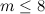$m\leq 8$, the following table summarises the known results on the spectrum problem for trees with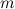$m$ edges. The graphs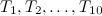$T_1,T_2,\ldots,T_{10}$ referenced in the table are shown in Figure 1. An explanation of the sources of these results is given in .

Table 1$m$ Spectrum for trees with$m$ edges Exceptions ($T_1,T_2,\ldots,T_{10}$ as in Figure 1)$1$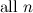${\rm all \ }n$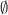$\emptyset$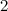$2$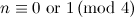$n \equiv 0 {\rm \ or \ } 1 \,({\rm mod \ }4)$$\emptyset$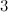$3$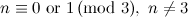$n \equiv 0 {\rm \ or \ } 1 \,({\rm mod \ }3),\; n\neq 3$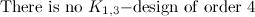${\rm There \ is \ no \ }K_{1,3}{\rm -design \ of \ order \ }4$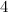$4$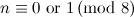$n \equiv 0 {\rm \ or \ } 1 \,({\rm mod \ }8)$$\emptyset$$5$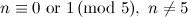$n \equiv 0 {\rm \ or \ } 1 \,({\rm mod \ }5),\;n\neq 5$${\rm There \ is \ no \ }K_{1,5}{\rm -design \ of \ order \ }6.$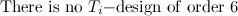${\rm There \ is \ no \ }T_{i}{\rm -design \ of \ order \ }6$${\rm for \ }i\in\{1,2\}.$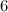$6$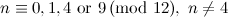$n \equiv 0,1,4 {\rm \ or \ } 9 \,({\rm mod \ }12),\;n\neq 4$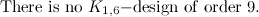${\rm There \ is \ no \ }K_{1,6}{\rm -design \ of \ order \ }9.$$7$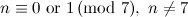$n \equiv 0 {\rm \ or \ } 1 \,({\rm mod \ }7),\;n\neq 7$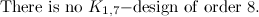${\rm There \ is \ no \ }K_{1,7}{\rm -design \ of \ order \ }8.$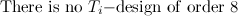${\rm There \ is \ no \ }T_{i}{\rm -design \ of \ order \ }8$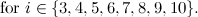${\rm for \ }i\in\{3,4,5,6,7,8,9,10\}.$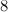$8$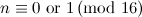$n \equiv 0 {\rm \ or \ } 1 \,({\rm mod \ }16)$$\emptyset$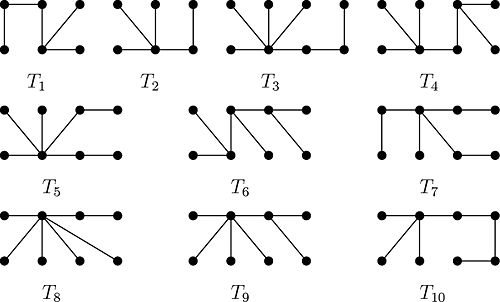The next five theorems provide spectrum results for other families of trees.

Theorem 1

Let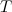$T$ be a tree belonging to one of the following families.

• Trees with at most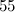$55$ vertices .
• Trees with at most$4$ endvertices .
• Trees of diameter at most$5$ , .
• Symmetric trees .

Then there exists a$T$-design of order$2|E(T)| + 1$.

Theorem 2

Let$T$ be a tree belonging to one of the following families.

• Trees with at most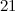$21$ vertices .
• Trees of diameter at most$5$ .
• Symmetric trees of diameter$4$ .
• Caterpillars .
• Comets .

Then there exists a$T$-design of order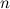$n$ for all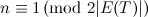$n \equiv 1 \,({\rm mod \ }2|E(T)|)$.

More results on labelings of trees, not encompassed by Theorem 1 and Theorem 2, can be found in Gallian's survey 'A dynamic survey of graph labeling' .

Theorem 3 

Let$T$ be a caterpillar or lobster with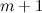$m+1$ vertices. If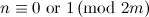$n \equiv 0 {\rm \ or \ }1 \,({\rm mod \ }2m)$, there exists a$T$-design of order$n$. Moreover, if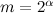$m = 2^{\alpha}$ for some integer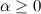$\alpha\geq 0$, then$n\equiv 0 {\rm \ or \ }1 \,({\rm mod \ }2m)$ is also necessary for existence.

Theorem 4 

Let$T$ be a tree with$m+1$ vertices. If$T$ contains a vertex of degree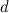$d$ such that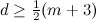$d \geq {1 \over 2} (m+3)$ then there does not exist a$T$-design of order$m+1$.

Theorem 5 

Let$T$ be a tree with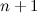$n+1$ vertices, let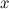$x$ be a vertex in$T$ and suppose either of the following holds.

• The graph obtained from$T$ by removing$x$ (and all the edges incident with$x$) has at least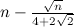$n-{\sqrt{n} \over 4+2\sqrt{2}}$ isolated vertices.
• For a non-negative integer$d$, the diameter of$T$ is at most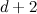$d+2$, and the graph obtained from$T$ by removing$x$ (and all the edges incident with$x$) has at least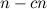$n-cn$ isolated vertices where$c=(\sqrt{1+(4+4d)^2}-4-4d)^2$.

Then there exists a$T$-design of order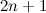$2n+1$.

## Notes

• The base of a graph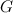$G$ is the graph obtained from$G$ by removing its endvertices.
• A caterpillar is a tree whose base is a path.
• A lobster is a tree whose base is a caterpillar.
• A comet is a graph obtained from a star by replacing each edge with a path of length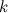$k$ for some fixed$k$.
• A tree is symmetric if it can be rooted so that any two vertices in the same level have the same degree.
• Numerous existence results for$G$-designs, in particular when$G$ is a tree, have been established under the guise of graph labelings, see  for more details.

## References

1. 1.0 1.1 1.2 Adams, P., Bryant, D., and Buchanan, M. A survey on the existence of G-designs, J. Combin. Des. 16, 373–410 (2008).
2. 2.0 2.1 Brankovic, L. and Rosa, A. (private communication).
3. 3.0 3.1 Dobson, E. Packing trees of bounded diameter into the complete graph, Australas. J. Combin. 37, 89–100 (2007).
4. 4.0 4.1 El-Zanati, S. I., Kenig, M. J., and Vanden Eynden, C. Near α-labelings of bipartite graphs, Australas. J. Combin. 21, 275–285 (2000).
5. 5.0 5.1 5.2 El-Zanati, S. I., Vanden Eynden, C., and Punnim, N. On the cyclic decomposition of complete graphs into bipartite graphs, Australas. J. Combin. 24, 209–219 (2001).
6. 6.0 6.1 Gallian, J. A. A dynamic survey of graph labeling, Electron. J. Combin. 5, i (1998).
7. 7.0 7.1 Grannell, M. J., Griggs, T. S., and Holroyd, F. C. Modular gracious labellings of trees, Discrete Math. 231, 199–219 (2001).
8. 8.0 8.1 Hrnčiar, P. and Haviar, A. All trees of diameter five are graceful, Discrete Math. 233, 133–150 (2001).
9. 9.0 9.1 Huang, C., Kotzig, A., and Rosa, A. Further results on tree labellings, Utilitas Math. 21, 31–48 (1982).
10. 10.0 10.1 10.2 Huang, C. and Rosa, A. Decomposition of complete graphs into trees, Ars Combin. 5, 23–63 (1978).
11. 11.0 11.1 Poljak, S. and Sûra, M. An algorithm for graceful labelling of a class of symmetrical trees, Ars Combin. 14, 57–66 (1982).
12. 12.0 12.1 Rosa, A. On certain valuations of the vertices of a graph, Theory of Graphs (Internat. Sympos., Rome, 1966), Gordon and Breach, New York, 349–355 (1967).
13. 13.0 13.1 Zhao, S. L. All trees of diameter four are graceful, Ann. New York Acad. Sci. Graph theory and its applications: East and West (Jinan, 1986), New York Acad. Sci. New York, 700–706 (1989).